# Double probability

The probability of success of the planned action is 60%. What is the probability that we will achieve success at least once if this action is repeated twice?

p =  0.84

### Step-by-step explanation: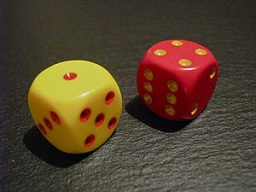Did you find an error or inaccuracy? Feel free to write us. Thank you!Tips to related online calculators
Would you like to compute count of combinations?

## Related math problems and questions:

• The vaccination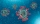The vaccination coverage of the population is 80%. Unvaccinated make up 60% of all infected. What percentage are unvaccinated more likely to be infected?
• Alarm systems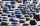What is the probability that at least one alarm system will signal the theft of a motor vehicle when the efficiency of the first system is 90% and of the independent second system 80%?
• The universityAt a certain university, 25% of students are in the business faculty. Of the students in the business faculty, 66% are males. However, only 52% of all students at the university are male. a. What is the probability that a student selected at random in the
• Utopia IslandThe probability of disease A on the island of Utopia is 40%. The probability of occurrence among the men of this island, which make up 60% of all the population (the rest are women), is 50%. What is the probability of occurrence of A disease among women o
• Two doctorsDoctor A will determine the correct diagnosis with a probability of 93% and doctor B with a probability of 79%. Calculate the probability of proper diagnosis if both doctors diagnose the patient.
• Probability of intersectionThree students have a probability of 0.7,0.5 and 0.4 to graduated from university respectively. What is the probability that at least one of them will be graduated?
• RecordsRecords indicate 90% error-free. If 8 records are randomly selected, what is the probability that at least 2 records have no errors?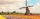How large must the group of people be so that the probability that two people have a birthday on the same day of the year is greater than 90%?
• Sick days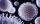In Canada, there are typically 261 working days per year. If there is a 4.9% chance that an employee takes a sick day. .. what is the probability an employee will use 17 OR MORE sick days in a year?
• MasteringThe student masters the subject matter for the exam in Czech to 98%, from Math to 86% and from Economics to 71%. What is the probability that he will fail from Math and from others will succeed?
• Left handedIt is known that 25% of the population is left-handed. What is the probability that there is a maximum of three left-handers at a seminar where there are 30 participants?
• Percents from percentHow much is 13% of 20% of 500 greater than 8% of 14% of 200?
• Dices throwsWhat is the probability that the two throws of the dice: a) Six falls even once b) Six will fall at least once
• ConcentrationHow many grams of 65% and 50% acid is to be mixed to give 240 g acid with a concentration of 60%?
• ShootersIn army regiment are six shooters. The first shooter target hit with a probability of 49%, next with 75%, 41%, 20%, 34%, 63%. Calculate the probability of target hit when shooting all at once.
• Alcohol mixingHow much 55% alcohol we must pour into 1500 g 80% alcohol to form a 60% alcohol? How much 60% alcohol created?
• Class - boys and girls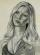In the class are 60% boys and 40% girls. Long hair has 10% boys and 80% girls. a) What is the probability that a randomly chosen person has long hair? b) The selected person has long hair. What is the probability that it is a girl?